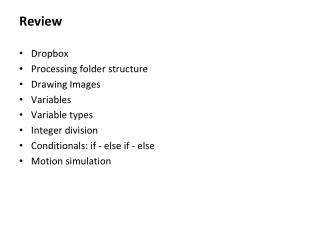Download PresentationReviewReview - PowerPoint PPT Presentation

Download PresentationReview
An Image/Link below is provided (as is) to download presentation

Download Policy: Content on the Website is provided to you AS IS for your information and personal use and may not be sold / licensed / shared on other websites without getting consent from its author. While downloading, if for some reason you are not able to download a presentation, the publisher may have deleted the file from their server.

- - - - - - - - - - - - - - - - - - - - - - - - - - - E N D - - - - - - - - - - - - - - - - - - - - - - - - - - -
Presentation Transcript

1. Review Dropbox Processing folder structure Drawing Images Variables Variable types Integer division Conditionals: if - else if - else Motion simulation

2. Expressions • Collections of data values and variables related by operators and function calls, and grouped by parentheses. • Expressions are automatically evaluated and replaced by the final evaluated value. • Expressions can be assigned to variables using “=“ • Expression is always on right • Variable name is always on left variable_name=expression;

3. Some Built-in Mathematical Functions sin(x), cos(x), tan(x), asin(x), … abs(x), exp(x), pow(x, y), log(x), sqrt(x), … max(x1, x2), min(x1, x2), floor(x), ceil(x), … dist(x1, y1, x2, y2) -> distance between two points norm(value, low, high) -> normalizes a value to [0-1] … and many more, all of which can be included in an expression.

4. Evaluating Expressions 1 + 2 pow(sin(x),2) + pow(cos(x),2) == 1.0 max(1, 2, 3) >= 2 floor(2.9) == ceil(1.8)

5. Iteration Repetition of a program block • Iterate when a block of code is to repeated multiple times. Options • The while-loop • The for-loop

6. Iteration: while-loop while ( boolean_expression ) { statements; // continue; // break; } • Statements are repeatedly executed while the boolean expression remains true; • To break out of a while loop, call break; • To stop execution of statements and start again, call continue; • All iterations can be written as while-loops.

7. void setup() { size(500, 500); smooth(); float diameter = 500.0; while ( diameter > 1.0 ) { ellipse( 250, 250, diameter, diameter); diameter = diameter * 0.9; } } void draw() { } What does this do? void setup() { size(500, 500); smooth(); float diameter = 500.0; while ( true ) { ellipse( 250, 250, diameter, diameter); diameter = diameter * 0.9; if (diameter <= 1.0 ) break; } } void draw() { }

8. An aside … Operators +, -, *, / and … i++;equivalent to i = i + 1; i += 2;equivalent toi = i + 2; i--;equivalent toi = i – 1; i -= 3;equivalent toi = i - 3; i *= 2;equivalent toi = i * 2; i /= 4;equivalent toi = i / 4; i % 3; the remainder after i is divided by 3 (modulo)

9. Iteration: for-loop for ( initialization; continuation_test; increment ) { statements; // continue; // break; } • A kind of iteration construct • initialization, continuation test and increment commands are part of statement • To break out of a while loop, call break; • To stop execution of statements and start again, call continue;

10. for (int i = 0; i < 10; i++ ){ print( i ); } println(); for (int i = 0; i < 10; i++ ) { if ( i % 2 == 1 ) continue; print( i ); } println();

11. void setup() { size(500, 500); smooth(); float diameter = 500; while ( diameter > 1 ) { ellipse( 250, 250, diameter, diameter); diameter = diameter – 10; } } void draw() { } void setup() { size(500, 500); smooth(); for (float diameter = 500; diameter > 1; diameter -= 10 ) { ellipse( 250, 250, diameter, diameter); } } void draw() { }